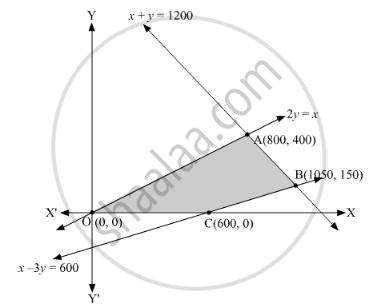# A Toy Company Manufactures Two Types of Dolls, A And B. Market Tests and Available Resources Have Indicated that the Combined Production - Mathematics

Sum

A toy company manufactures two types of dolls, A and B. Market tests and available resources have indicated that the combined production level should not exceed 1200 dolls per week and the demand for dolls of type B is at most half of that for dolls of type A. Further, the production level of dolls of type A can exceed three times the production of dolls of other type by at most 600 units. If the company makes profit of ₹ 12 and ₹ 16 per doll respectively on dolls A and B, how many of each should be produced weekly in order to maximise the profit?

#### Solution

Let $x$  units of doll A and $y$  units of doll B are manufactured to obtain the maximum profit.
The mathematical formulation of the above problem is as follows:
Maximize $Z = 12x + 16y$
Subject to

$x + y \leq 1200$

$y \leq \frac{x}{2}$

$x - 3y \leq 600$

$x, y \geq 0$The shaded region represents the set of feasible solutions.
The coordinates of the corner points of the feasible region are O(0,0), A(800,400), B(1050,150) and C(600,0).
$= 12\left( 0 \right) + 16\left( 0 \right) = 0$
The value of Z at A(800,400) $= 12\left( 800 \right) + 16\left( 400 \right) = 16000$  (Maximum)
The value of Z at B(1050,150) $= 12\left( 1050 \right) + 16\left( 150 \right) = 15000$
The value of Z at C(600,0)
$= 12\left( 600 \right) + 16\left( 0 \right) = 7200$
Therefore, 800 units of doll A and 400 units of doll B should be produced weekly to get the maximum profit of Rs 16000.

Concept: Different Types of Linear Programming Problems
Is there an error in this question or solution?

#### APPEARS IN

RD Sharma Class 12 Maths
Chapter 30 Linear programming
Exercise 30.4 | Q 46 | Page 56